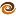# EmptyCrate

#### About Me | Contact Me | Training |ChaiScript.com | YouTube Channel

boost contains a preprocessor metaprogramming libary. What this means, simply, is that it is possible to write code which generates code. The full docs are here.

It is possible to do full LISP-like lambda calculus with the library. That’s not, however, where my interests lie. For me, the practical use is to automatically generate a set of templates which take N arguments and let the compiler generate all of the N iterations for you.

Here is a simple example. Say you want a set of template functions which convert any set of objects into their string representations using the boost::lexical_cast library.

``````#include <boost/preprocessor.hpp>
#define rep(z, n, text) \
a.push_back(lexical_cast<string>(x ## n);
#ifndef BOOST_PP_IS_ITERATING
# ifndef CONVERTOBJECTS_HPP
# define CONVERTOBJECTS_HPP
//0 object case

vector<string> convertObjsToStrings() {
return vector<string>();
}

#define BOOST_PP_ITERATION_LIMITS ( 1, 3)
#define BOOST_PP_FILENAME_1 "convertobjects.h"
#include BOOST_PP_ITERATE()
# endif
#else
# define n BOOST_PP_ITERATION()

template < BOOST_PP_ENUM_PARAMS(n, class A) >

vector<string> convertObjsToStrings(
BOOST_PP_ENUM_BINARY_PARAMS(n, A, x )
)
{
vector<string> a;
BOOST_PP_REPEAT(n, rep, ~)
return a;
}

#endif
``````

In the above code we have a few very simple concepts. First, we see that the code is going to iterate 3 times with the line: #define BOOST_PP_ITERATION_LIMITS ( 1, 3).

Second, we see that the header file includes itself with the line: #define BOOST_PP_FILENAME_1 “convertobjects.h”.

Third, we accomplish the real work by iterating the rep macro define at the top of the file with the line: BOOST_PP_REPEAT(n, rep, ~)

This probably seems pretty abstract if you are anything like me. Below is the output from the C++ preprocessor, to show you the code that is generated:

``````template < class A0 >
vector<string> convertObjsToStrings( A0 x0 )
{
vector<string> a;
a.push_back(lexical_cast<string>(x0);
return a;
}

template < class A0 , class A1 >

vector<string> convertObjsToStrings( A0 x0 , A1 x1 )
{
vector<string> a;
a.push_back(lexical_cast<string>(x0);
a.push_back(lexical_cast<string>(x1);
return a;
}

template < class A0 , class A1 , class A2 >
vector<string> convertObjsToStrings( A0 x0 , A1 x1 ,
A2 x2 )
{
vector<string> a;
a.push_back(lexical_cast<string>(x0);
a.push_back(lexical_cast<string>(x1);
a.push_back(lexical_cast<string>(x2);
return a;
}
``````

Of course you can increase the number of allowed parameters pretty high, I think up to 256 using the BOOST_PP_LIMIT_REPEAT macro.

My Videos

Related